# NCERT Solutions for Class 9 Science Forces and Laws of Motion part 2## myCBSEguide App

CBSE, NCERT, JEE Main, NEET-UG, NDA, Exam Papers, Question Bank, NCERT Solutions, Exemplars, Revision Notes, Free Videos, MCQ Tests & more.

NCERT solutions for Science Forces and Laws of Motion## NCERT Class 9 Science Chapter wise Solutions

• 01 –  Matter in Our Surroundings
• 02 – Is Mattter Around us Pure
• 03 – Atoms and Molecules
• 04 – Structure of the Atom
• 05 – The Fundamental Unit of Life
• 06 – Tissues
• 07 – Diversity in Living Organisms
• 08 – Motion
• 09 – Force and Laws of Motion
• 10 – Gravitation
• 11 – Word and Energy
• 12 – Sound
• 13 – Why Do We Fall Ill
• 14 – Natural Resources
• 15 – Improvement in Food Resources

## NCERT Solutions for Class 9 Science Forces and Laws of Motion part 2

###### 1. An object experiences a net zero external unbalanced force. Is it possible for the object to be travelling with a non-zero velocity? If yes, state the conditions that must be placed on the magnitude and direction of the velocity. If no, provide a reason.

Ans. No, it is not possible for the object to be travelling with a non-zero velocity if an object experiences a net zero external unbalanced force since unbalanced forces cannot be equal to zero.

###### 2. When a carpet is beaten with a stick, dust comes out of it. Explain.

Ans. When a carpet is beaten with a stick, dust comes out of it because carpet fibres vibrate in forward and backward direction as carpet is beaten but the loosely bound dust particles due to inertia remain at rest and so they come out.

###### 3. Why is it advised to tie any luggage kept on the roof of a bus with a rope?

Ans. It is advised to tie any luggage kept on the roof of a bus with a rope because when bus moves the luggage also gets moving with the velocity same as that of the bus and in the same direction but when bus changes direction or deaccelerates, due to inertia of motion luggage moves in the same direction and may get thrown away from roof of buses.

###### 4. A batsman hits a cricket ball which then rolls on a level ground. After covering a short distance, the ball comes to rest. The ball slows to a stop because

(a) the batsman did not hit the ball hard enough.

(b) velocity is proportional to the force exerted on the ball.

(c) there is a force on the ball opposing the motion.

(d) there is no unbalanced force on the ball, so the ball would want to come to rest.

Ans. (c) there is a force on the ball opposing the motion.

###### 5. A truck starts from rest and rolls down a hill with a constant acceleration. It travels a distance of 400 m in 20 s. Find its acceleration. Find the force acting on it if its mass is 7 metric tonnes (Hint: 1 metric tonne = 1000 kg.)

Ans. According to the question,

initial velocity of truck (u) = 0

distance = s= 400 m and time = 20 s

mass of truck = 7metric tones = 7000kg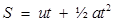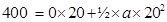400 =0 + 200a

400 = 200a

a = 400/200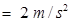therefore,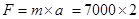= 14000 N

NCERT Solutions for Class 9 Science Forces and Laws of Motion part 2

###### 6. A stone of 1 kg is thrown with a velocity of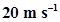across the frozen surface of a lake and comes to rest after travelling a distance of 50 m. What is the force of friction between the stone and the ice?

Ans. since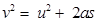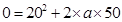(object comes to rest so v=0)

-100a = 400

a = 400/-100 =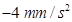therefore, the force of friction between the stone and the ice

= -4 N

NCERT Solutions for Class 9 Science Forces and Laws of Motion part 2

###### 7. A 8000 kg engine pulls a train of 5 wagons, each of 2000 kg, along a horizontal track. If the engine exerts a force of 40000 N and the track offers a friction force of 5000 N, then calculate:

(a) the net accelerating force;

(b) the acceleration of the train; and

(c) the force of wagon 1 on wagon 2.

Ans. (a) The net accelerating force = Force exerted by engine – frictional force of track = 40000 – 5000 = 35000 N

(b) the acceleration of the train = a = F/m =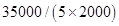= 35000/10000 =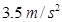(c) the force of wagon 1 on wagon 2

Wagon 1 will have to exert force on all 4 wagons next to it

so mass of other 4 wagons =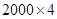= 8000 kg

F = ma =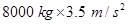= 28000 N

###### 8. An automobile vehicle has a mass of 1500 kg. What must be the force between the vehicle and road if the vehicle is to be stopped with a negative acceleration of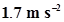?

Ans. since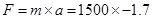= -2550 N (negative sign symbolises acceleration in opposite direction)

###### 9. What is the momentum of an object of mass m, moving with a velocity v?

(a)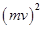(b)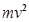(c)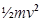(d) mv

Ans. (d) mv

###### 10. Using a horizontal force of 200 N, we intend to move a wooden cabinet across a floor at a constant velocity. What is the friction force that will be exerted on the cabinet?

Ans. 200 N

NCERT Solutions for Class 9 Science Forces and Laws of Motion part 2

###### 11. Two objects, each of mass 1.5 kg, are moving in the same straight line but in opposite directions. The velocity of each object is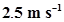before the collision during which they stick together. What will be the velocity of the combined object after collision?

Ans. Momentum before collision took place =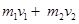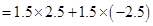= 0

Since the objects stick together after collision hence

momentum after collision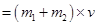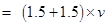= 3v

momentum before collision = momentum after collision

0 = 3v, v= 0/3 = 0

the velocity of the combined object after collision (v)= 0

###### 12. According to the third law of motion when we push on an object, the object pushes back on us with an equal and opposite force. If the object is a massive truck parked along the roadside, it will probably not move. A student justifies this by answering that the two opposite and equal forces cancel each other. Comment on this logic and explain why the truck does not move.

Ans. According to the third law of motion when we push on an object, the object pushes back on us with an equal and opposite force result is the two opposite and equal forces cancel each other but when one of these forces is bigger than inertia so the object moves in the direction of force applied. As this student explains the truck is massive so the force applied cannot overcome force caused by inertia. Therefore, the truck does not move.

NCERT Solutions for Class 9 Science Forces and Laws of Motion part 2

###### 13. A hockey ball of mass 200 g travelling at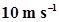is struck by a hockey stick so as to return it along its original path with a velocity at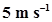. Calculate the change of momentum occurred in the motion of the hockey ball by the force applied by the hockey stick.

Ans. mass of hockey ball = 200g = 0.2 kg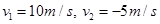(return velocity)

initial momentum of hockey ball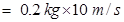= 2 kg m/s

final momentum of hockey ball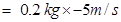= -1 kg m/s

change in momentum of hockey ball = 2 – (-1) = 2 + 1 = 3 kg m/s

###### NCERT Solutions for Class 9 Science Forces and Laws of Motion part 2

14. A bullet of mass 10 g travelling horizontally with a velocity of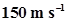strikes a stationary wooden block and comes to rest in 0.03 s. Calculate the distance of penetration of the bullet into the block. Also calculate the magnitude of the force exerted by the wooden block on the bullet.

Ans. v =u + at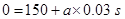a = -150/0.03 =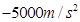the distance of penetration of the bullet into the block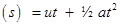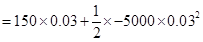= 4.5 – 2.25

= 2.25 m

the magnitude of the force exerted by the wooden block on the bullet

m = 10g = 0.01kg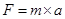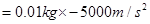= -50 N

NCERT Solutions for Class 9 Science Forces and Laws of Motion part 2

###### 15. An object of mass 1 kg travelling in a straight line with a velocity ofcollides with, and sticks to, a stationary wooden block of mass 5 kg. Then they both move off together in the same straight line. Calculate the total momentum just before the impact and just after the impact. Also, calculate the velocity of the combined object.

Ans. Wooden block is stationery (at rest) so its velocity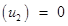mass of combined object is = 1 kg + 5 kg = 6 kg

total momentum before the impact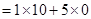= 10 kg m/s

law of conservation of momentum:

total momentum just before the impact = total momentum after the impact= 10 kg m/s

therefore, the velocity of the combined object: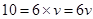v = 10/6 = 1.67 m/s

NCERT Solutions for Class 9 Science Forces and Laws of Motion part 2

###### 16. An object of mass 100 kg is accelerated uniformly from a velocity ofto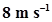in 6 s. Calculate the initial and final momentum of the object. Also, find the magnitude of the force exerted on the object.

Ans. Initial momentum of the object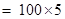= 500 kg m/s

Final momentum of the object =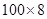= 800 kg m/s

since v = u + at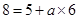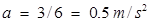since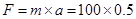= 50 N

NCERT Solutions for Class 9 Science Forces and Laws of Motion part 2

###### 17. Akhtar, Kiran and Rahul were riding in a motorcar that was moving with a high velocity on an expressway when an insect hit the windshield and got stuck on the windscreen. Akhtar and Kiran started pondering over the situation. Kiran suggested that the insect suffered a greater change in momentum as compared to the change in momentum of the motorcar (because the change in the velocity of the insect was much more than that of the motorcar). Akhtar said that since the motorcar was moving with a larger velocity, it exerted a larger force on the insect. And as a result the insect died. Rahul while putting an entirely new explanation said that both the motorcar and the insect experienced the same force and a change in their momentum. Comment on these suggestions.

Ans. Since the mass of insect is negligible in comparison to the mass of motorcar therefore there will be no any change in the momentum of motorcar.

###### 18. How much momentum will a dumb-bell of mass 10 kg transfer to the floor if it falls from a height of 80 cm? Take its downward acceleration to be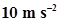.

Ans. height from which dumb bell falls = 80 cm = 0.8 m

since we know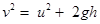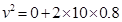= 16

v =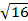v = 4 m/s

momentum = mv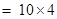= 40 kg m/s

## NCERT Solutions for Class 9 Science

NCERT Solutions Class 9 Science PDF (Download) Free from myCBSEguide app and myCBSEguide website. Ncert solution class 9 Science includes text book solutions .NCERT Solutions for CBSE Class 9 Science have total 15 chapters. 9 Science NCERT Solutions in PDF for free Download on our website. Ncert Science class 9 solutions PDF and Science ncert class 9 PDF solutions with latest modifications and as per the latest CBSE syllabus are only available in myCBSEguide.

## CBSE app for Class 9

To download NCERT Solutions for class 9 Science, Computer Science, Home Science,Hindi ,English, Maths Social Science do check myCBSEguide app or website. myCBSEguide provides sample papers with solution, test papers for chapter-wise practice, NCERT solutions, NCERT Exemplar solutions, quick revision notes for ready reference, CBSE guess papers and CBSE important question papers. Sample Paper all are made available through the best app for CBSE students and myCBSEguide website.### 1 thought on “NCERT Solutions for Class 9 Science Forces and Laws of Motion part 2”

1. amezing site